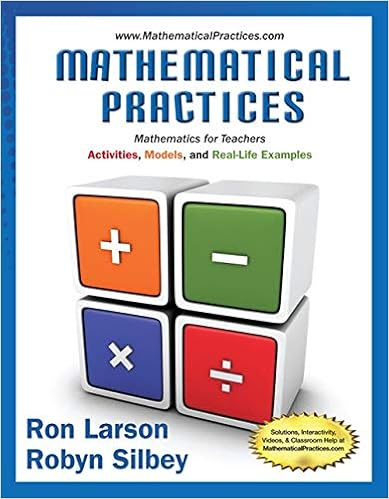# The nygen family wants to put a circular fence around

• Test Prep
• 46
• 99% (112) 111 out of 112 people found this document helpful

This preview shows page 36 - 39 out of 46 pages.

##### We have textbook solutions for you!
The document you are viewing contains questions related to this textbook.The document you are viewing contains questions related to this textbook.
Chapter 9 / Exercise 77
Mathematical Practices, Mathematics for Teachers: Activities, Models, and Real-Life Examples
LarsonExpert Verified
83) The Nygen family wants to put a circular fence around their pool. They want it to be exactly 12 feet from the center of the pool. How many feet of fencing do they need to buy? @ C 2 (12) <alt tag = C equals 2 pi times 12> C = 75.4 ft 76 ft a. 75 *b. 76 c. 24 d. 48
84) If the circumference of a circle is 22 in, find the diameter. @ 22 d   <alt tag = 22 equals pi d> d = 7 in
85) Use the illustration below to calculate the circumference of the circle
@ To find the circumference, first find the diameter. To find the diameter, use the Pythagorean Theorem. d
86) Use the illustration below to calculate the circumference of the circle.
##### We have textbook solutions for you!
The document you are viewing contains questions related to this textbook.The document you are viewing contains questions related to this textbook.
Chapter 9 / Exercise 77
Mathematical Practices, Mathematics for Teachers: Activities, Models, and Real-Life Examples
LarsonExpert Verified
<alt tag = circle with radius 4> @ C 2 (4) <alt tag = C equals 2 pi times 4> C = 25.1 *a. 25.1 b. 12.56 c. 6.29 d. 50.27
87) Miley’s dog, Ella loves to play outside. Ella’s 11.5 ft leash is tied to a stake in the ground. she walks around in a circle, how far did she walk? If
Objective 12: Students will be able to find the circumference of a circle Display 1 Type: E 88) Use the illustration below to calculate the circumference of the circle
a. To find the circumference, first find the diameter. To find the diameter, use the Pythagorean Theorem. d
Type: E 89) The diameter of the Earth is approximately 7,957.7 miles. Find the circumference of the Earth to the nearest mile.
Objective 13: Students will be able to find the area of the circle Display 2 90) Miley’s dog, Ella loves to play outside. Ella’s 15 ft leash is tied to a stake in the ground. How much area does she have to play? 2
91) The size of a bicycle is determined by the diameter of the wheel. You want a 21-in bicycle for your birthday. What is the area of one of the wheels?
•••